Next: Centrifugal Acceleration Up: Rotating Reference Frames Previous: Introduction

# Rotating Reference Frames

Suppose that a given object has position vectorin some non-rotating inertial reference frame. Let us observe the motion of this object in a non-inertial reference frame which rotates with constant angular velocity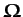about an axis passing through the origin of the inertial frame. Suppose, first of all, that our object appears stationary in the rotating reference frame. Hence, in the non-rotating frame, the object's position vectorwill appear to precess about the origin with angular velocity. It follows, from Equation (A.1309), that in the non-rotating reference frame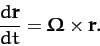(406)

Suppose, now, that our object appears to move in the rotating reference frame with instantaneous velocity. It is fairly obvious that the appropriate generalization of the above equation is simply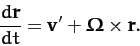(407)

Let andand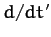denote apparent time derivatives in the non-rotating and rotating frames of reference, respectively. Since an object which is stationary in the rotating reference frame appears to move in the non-rotating frame, it is clear that. Writing the apparent velocity,, of our object in the rotating reference frame as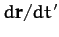, the above equation takes the form(408)

or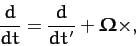(409)

sinceis a general position vector. Equation (409) expresses the relationship between apparent time derivatives in the non-rotating and rotating reference frames.

Operating on the general position vectorwith the time derivative (409), we get(410)

This equation relates the apparent velocity,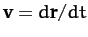, of an object with position vectorin the non-rotating reference frame to its apparent velocity,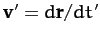, in the rotating reference frame.

Operating twice on the position vectorwith the time derivative (409), we obtain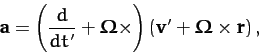(411)

or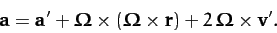(412)

This equation relates the apparent acceleration,, of an object with position vectorin the non-rotating reference frame to its apparent acceleration,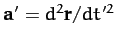, in the rotating reference frame.

Applying Newton's second law of motion in the inertial (i.e., non-rotating) reference frame, we obtain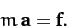(413)

Here,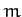is the mass of our object, and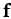is the (non-fictitious) force acting on it. Note that these quantities are the same in both reference frames. Making use of Equation (412), the apparent equation of motion of our object in the rotating reference frame takes the form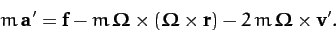(414)

The last two terms in the above equation are so-called fictitious forces''. Such forces are always needed to account for motion observed in non-inertial reference frames. Note that fictitious forces can always be distinguished from non-fictitious forces in Newtonian dynamics because the former have no associated reactions. Let us now investigate the two fictitious forces appearing in Equation (414).Next: Centrifugal Acceleration Up: Rotating Reference Frames Previous: Introduction
Richard Fitzpatrick 2011-03-31